# IIT Physics Sample Paper 1

### Rotational Kinematics & Dynamics

1. A uniform thin rod AB of mass 3m and length 2a is free to rotate in a horizontal plane about a smooth fixed vertical axis which passes through the midpoint O of the rod. Two small smooth rings each of mass m are free to slide on the rod. At time t=0 the rings are on the opposite side of O and are at a distance a/2 from O. The rod is then given an initial angular velocity 2Ög/a, the rings being initially at rest relative to the rod.
1. Show that when the rings are about to slip off the rod its angular velocity is Ög/a.
2. Find the speed of either ring at this instant.
3. If the point O is at a height a above the horizontal plane show that the distance between the points where the ring strikes the plane is 2aÖ(6 + 2Ö3).
2. Two particles m1 and m2 are connected by a light elastic string of natural length lo and are placed on a smooth horizontal table with separation between m1 and m2 as lo. m2 is projected with a velocity vo along the table at right angles to the string. Show that the max length l of the particle is given by: l2(l-lo)=m1m2vo2(l+lo)/k(m1+m2) where k is force const of string
3.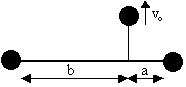Two equal particles are attached to the end of a light rod and a 3rd equal particle is connected by an inextensible string to a point on the rod at distance a and b from its ends. The latter particle is projected at right angles to the rod with a velocity vo. Show that when the string becomes taut the velocity of the particle is changed to v1=vo(a2+b2)/2(a2+ab+b2) and that the rod begins to turn with angular velocity (a-b/a2+b2)v1.
4. A rod of length 2a and mass M is in motion in a horizontal plane with speed u at right angle to its length when it collides with a small elastic sphere of equal mass whose center lies in the same plane. If the sphere is free to move, Prove that angular velocity acquired by the rod cannot exceed (1+e)uÖ6/4a.
5. A cube rests on a rough plane of inclination q (< p/4) with 2 of its upper and two of its lower edges horizontal. A rope is attached to the midpoint of its upper most edge and is pulled parallel to the greatest slope of the plane. Show that it will be impossible to drag the cube without upsetting it if the coefficient of friction exceeds (1-tan q)/2
6.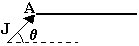A uniform rod of length 2a, mass m is set in motion by a sudden blow J acting at A and inclined at q to rod as shown. Find velocity of A of rod immediately after the impact and Prove that energy communicated is J2(1+3sin2q)/2m.
7.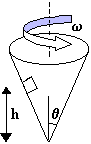A small block of mass m is placed inside a hollow cone rotating about a vertical axis with angular velocity w as in the figure. The semivertical angle of the cone is q and the coefficient of friction between the cone and the block is m. If the block is to remain at a constant height h above the apex of the cone, what are the maximum and minimum values of w?
8.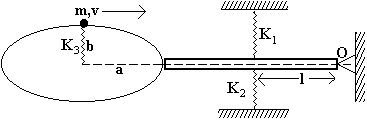The shown arrangement is in a horizontal plane. The particle with mass m is restricted to move in an elliptical orbit with shown dimension. The particle has velocity v at the shown position towards right. Length of rod is 2l. The coefficient of restitution between the particle and the rod is 1/3. The moment of inertia of rod is I, about O.
1. Find the locus of mid-point of spring of force constant K3.
2. Find the frequency of the rod.
3. What would be the maximum angular displacement of the oscillating system.
(Assume only one collision)
9.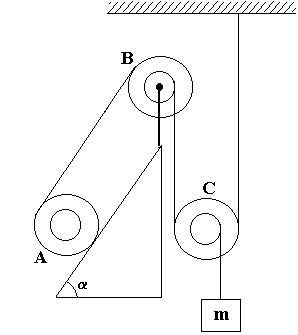The system shown is initially at rest. Find the acceleration of the bobbins A and mass m. Assume that A can roll without slipping along the incline. Given that the MI about instantaneous axis of rotation of bobbins A,B,C is IA, IB, IC. Assume their masses to be mA, mB, mc and internal and external radii to be r and R.IIT JEE - HomeWebsites of IITJEE SyllabusIIT Question PapersBooks For IITIIT JEE 2008IIT JEE 2007IIT JEE 2006IIT JEE 2005IIT CoursesSeats ReservationEligibility CriteriaAnalysis of IIT JEEIIT AlumniIIT JEE TipsIIT Articles